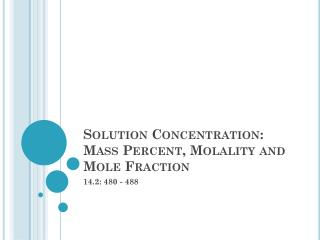Download PresentationSolution Concentration: Mass Percent, Molality and Mole Fraction

Solution Concentration: Mass Percent, Molality and Mole Fraction - PowerPoint PPT Presentation

Solution Concentration: Mass Percent, Molality and Mole Fraction. 14.2: 480 - 488. Main Idea. Concentration can be expressed in terms of percent or in terms of moles. Methods for Showing Concentration. Molarity Percent by mass Percent by Volume Molality Mole Fractions.I am the owner, or an agent authorized to act on behalf of the owner, of the copyrighted work described.
Download PresentationSolution Concentration: Mass Percent, Molality and Mole Fraction

Download Policy: Content on the Website is provided to you AS IS for your information and personal use and may not be sold / licensed / shared on other websites without getting consent from its author.While downloading, if for some reason you are not able to download a presentation, the publisher may have deleted the file from their server.

- - - - - - - - - - - - - - - - - - - - - - - - - - E N D - - - - - - - - - - - - - - - - - - - - - - - - - -
Presentation Transcript
1. Solution Concentration: Mass Percent, Molality and Mole Fraction 14.2: 480 - 488

2. Main Idea • Concentration can be expressed in terms of percent or in terms of moles

3. Methods for Showing Concentration • Molarity • Percent by mass • Percent by Volume • Molality • Mole Fractions

4. Percent Solution • Two percentage methods to show how concentrated a solution is: • Percent by volume = volume of solute x 100% volume of solution • Percent by (m/v) = mass of solute x 100% solution volume(mL)

5. Percent Solution • Examples: • If 10. mL of pure acetone is diluted with water to a total solution volume of 200. mL, what is the percent by volume of the acetone solution? Percent by volume = volume of solute x 100% volume of solution Percent by volume = 10 x 100% = 50.% 200

6. Percent Solution • Examples: Calculate the grams of solute needed to make 250. mL of a 0.10% MgSO4 solution. Percent by (m/v) = mass of solute x 100% volume of solution (mL) = 0.10%= ___?__ x 100% 250mL ? = 0.25g MgSO4

7. Molality • Another way to express solution concentration is Molality (m)

8. Examples • How many grams of sodium fluoride are needed to prepare a 0.40m NaF solution that contains 750.0g of water?

9. Examples • Calculate the molality of a solution prepared by dissolving 10.0g of NaCl in 600.g of water. m = 0.290 m

10. Mole Fraction • Solution concentration can also be expressed by using the mole fraction Mol solute Mole Fraction Total moles in solution

11. Examples • What is the mole fraction of each component in a solution made by mixing 1.25 mol ethylene glycol and 4.00 mol water?

12. Examples What is the mole fraction of each component in a solution made by mixing 300.g of ethanol (C2H5OH) and 500.g water? Moles of ethanol = 6.51mol Moles of water = 27.8mol Mole fraction of ethanol = 6.51/(27.8+6.51) =0.190 Mole fraction of water = 27.8/(27.8+6.51) =0.810

13. Honors: Before you go… • Calculate the mass of calcium cyanide needed to make a 0.25m calcium cyanide solution using 450g of water.